# PSEB 9th Class Science Important Questions Chapter 3 Atoms and Molecules

Punjab State Board PSEB 9th Class Science Important Questions Chapter 3 Atoms and Molecules Important Questions and Answers.

## PSEB 9th Class Science Important Questions Chapter 3 Atoms and Molecules

Question 1.
(a) Write the important postulates of the Dalton’s Atomic Theory.
(b) Give four drawbacks of Dalton’s Atomic Theory.
(a) Dalton’s atomic theory was given by John Dalton in 1808 to e×plain the nature of an atom and the chemical combination in the atoms. The main points of the theory are:

1. All matter is made up of a large number of e×tremely small particles called atoms.
2. An atom cannot be further divided.
3. Atoms are of different kinds. There are as many kinds of atoms as are of elements.
4. Atoms of a particular element are identical in all respects i.e. they have same mass and similar properties.
5. Atoms of the different elements are different i.e. they have different masses and properties.
6. Two or more atoms of the same or different elements combine to form compound atoms or molecules of compounds.
7. The number and the nature of atoms in a molecule always remains the same.
8. Atoms of the different elements combine in a simple whole number but fi×ed ratios to form compound atoms (now called molecules).
9. Atoms of the same element can combine to form two or more compounds.
10. Atom can neither be created nor be destroyed.

(b) Drawbacks of Dalton’s Atomic Theory:

1. Atom is no longer indivisible.
2. Atoms of the same element may not be always identical.
3. Atoms of different elements may have same atomic masses.
4. It does not e×plain the cause of chemical combination.Question 2.
How to write a chemical formula of an ionic compound from its radicals?
While writing the formula of an ionic compound, the following steps are used :

1. Write down the symbol or formula of positive ion to the left and the symbol or formula of the negative ion to the right.
2. Shift the valency of positive ion to the lower right of the negative ion and the valency of negative ion to the lower right of the positive ion.
3. Take out the common factor if any.
4. The formula compound radical is enclosed in small brackets and subscript is put outside the bracket. The subscript 1 is omitted.

Example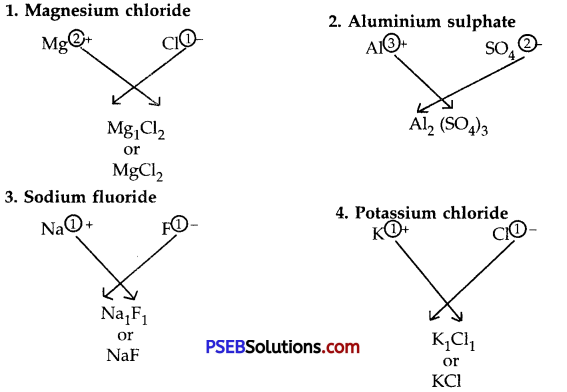Question 3.
How will you write the name of a binary molecular compound?
A covalent compound made up of two elements is called a binary molecular compound, e.g. water (H20).
While writing the name of a binary molecular compound, first write the name of the element whose symbol appears on the left hand side in the molecular formula and write down the name of other element. The name of the other element should end with ‘ide’. The prefi×es di, tri, tetra, penta etc. are used to indicate 2, 3, 4, 5…. atoms of an element.

Some examples are:

• Compound – Name
• CO – Carbon mono×ide
• CO2 – Carbon dio×ide
• ZnO – Zinc O×ide
• SO2 – Sulphur dio×ide
• PCl3 – Phosphorus trichloride
• PCl5 – Phosphorus pentachlorideQuestion 4.
Give one experiment to prove the truth of law of conservation of mass.
1. Prepare 5% solution of barium chloride and sodium sulphate in separate beaker.
2. Take a small amount of solution of barium chloride in a conical flask and a small amount of solution of sodium sulphate in an ignition tube.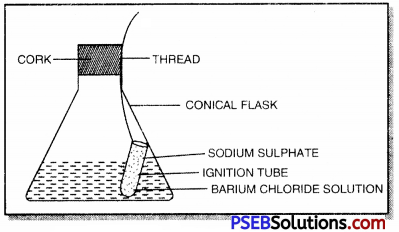3. Suspend the ignition tube carefully in the conical flask with a thread and cork it.
4. Weigh the flask carefully. To prove the law of conservation of mass
5. Tilt and swirl the flask such that the two solutions get mi×ed and react.
6. Cool the flask to room temperature and again weigh it. The weight remains unchanged showing the truth of the law.

Question 1.
Differentiate between an atom and a molecule.

 Atom Molecule 1. It is the smallest particle of an element which takes part in chemical reactions. It may or may not exist freely. 1. It is the smallest particle of a substance which can exist freely. 2. Generally it is highly reactive. 2. It is less reactive. 3. It is less stable. 3. It is more stable. 4. It can’t be sub-divided. 4. It can be sub-divided.

Question 2.
(a) What do you understand by the term chemical formula?
(b) What qualitative information is given by the formula NH3?
(a) Chemical formula. The symbolic representation of a molecule of a substance is called its chemical formula. It indicates the actual number of atoms of various elements present in one molecule of a substance.
(b) NH3 represents ammonia.

Question 3.
Write down the formulae of:
(a) Aluminium oxide
(b) Aluminium chloride
(c) Hydrogen sulphide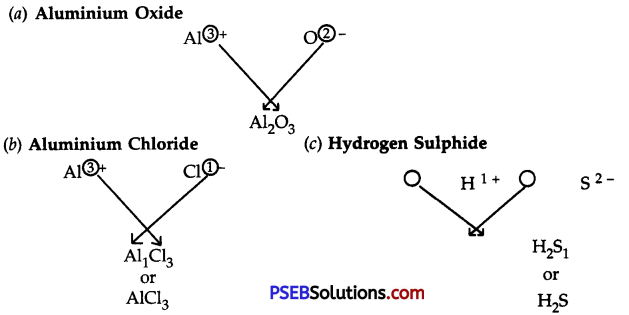Question 4.
Calculate the relative molecular masses of:
(a) Water (H2O)
(b) Nitric Acid (HNO3).
(a) Molecular mass of water (H2O) = 2 × 1 + 16 = 2 +16 = 18u.
(b) Molecular mass of nitric acid (HNO3) = 1 + 14 + 3 × 16 =1 + 14 + 48 = 63u.Question 5.
Write down the names of the compounds represented by the following formulae:

1. Na2CO3
2. MgCl2
3. Al2 (SO4)2
4. K2 S04
5. NiS04
6. KN03
7. CaC03

1. Sodium Carbonate
2. Magnesium Chloride
3. Aluminium Sulphate
4. Potassium Sulphate
5. Nickel Sulphate
6. Potassium Nitrate
7. Calcium Carbonate.

Question 6.
Work out the formula of:
1. Ammonia
2. Carbon dio×ide
3. Carbon tetrachloride.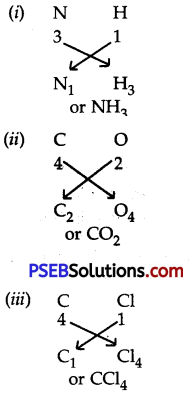Question 7.
Calculate the number of moles in:
1. 52 g of He
2. 12.044 × 1023 atoms of He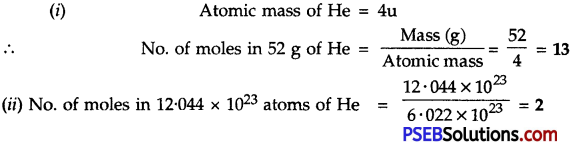Question 8.
Give one e×ample in each case;
(a) Diatomic molecule
(b) Triatomic molecule
(c) Monoatomic molecule
(a) Dihydrogen (H2)
(b) Ozone (03)
(c) Helium (He).

Question 9.
An element A has a charge of 3+. Write down the formulae of its:
(a) Chloride
(b) Sulphate
(c) Nitrate and
(d) Phosphate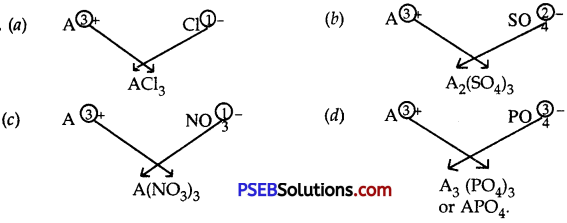Question 10.
Write the formulae and names of the compounds between:
1. Sodium and sulphate ions
2. Aluminium and chloride ions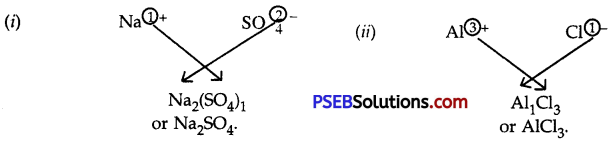Question 11.
Write the formulae of the compounds formed by:

1. Cr3+ and F
2. Pb2+ and $$\mathrm{SO}_{4}^{2-}$$
3. Mg2+ and S2-
4. $$\mathrm{NH}_{4}^{+}$$ and $$\mathrm{Cr2O}_{7}^{2-}$$
5. K+ and $$\mathrm{CrO}_{4}^{2-}$$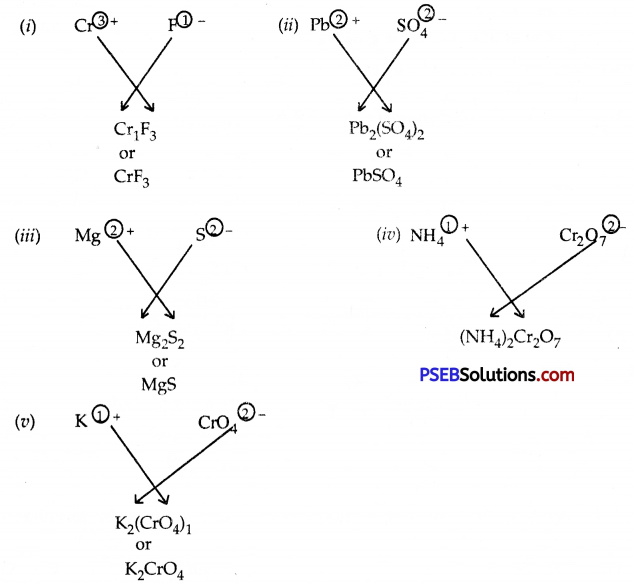Question 12.
Calculate the formula mass of Na2CO3.10H2O.
Formula mass of Na2,CO3.10H2O = 2 × 23 + 12 + 3 × 16 + 10 (2 × 1 + 16)
= 46 + 12 + 48 + 10 (2 + 16)
= 46 + 12 + 48 + 180
= 286 a.m.u.Question 13.
(a) What do you understand by trivial names of compounds?
(b) Give the chemical names of five such compounds and give their trivial names.
(a) The names of the compounds which do not follow any systematic rules are called trivial names or common names.
(b)

 Compound Chemical name Trivial name NH3 Nitrogen trihydride (AZANE) Ammonia NaCl Sodium chloride Common salt or Table salt H2O Hydrogen hydroxide (OXIDANE) Water NaOH Sodium hydroxide Caustic soda PH3 Phosphorous trihydride (PHOSPHANE) . Phosphine.

Question 14.
Calculate:
1. The number of atoms in 46 g of Na
2. No. of molecules in 8 g of O2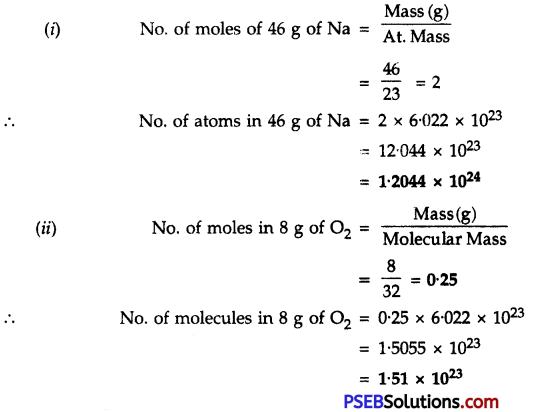Question 15.
An element × shows two valencies i.e. 2 and 4. Write the formulae of its o×ides.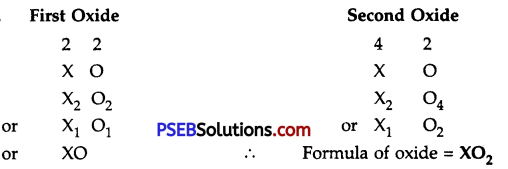∴ Formula of oxide – xO

Question 16.
Write the formulae of the following salts:

• Zinc carbonate
• Ammonium sulphate
• Barium chloride
• Sodium nitrate
• Potassium permanganate

• Name of the compound – Formula
• Zinc carbonate – ZnCO3
• Ammonium sulphate – (NH4)2SO4
• Barium chloride – BaCl2
• Sodium nitrate – NaN03
• Potassium permangante – KMnO4Question 17.
Write the names of the following compounds:

1. Al2(S04)3
2. Mg(HCO3)2
3. (NH4)2S
4. KMnO4
5. KClO3

Formula of the Compound Name

1. Al2(S04)3 – Aluminium sulphate
2. Mg(HC03)2 – Magnesium bicarbonate
3. (NH4)2 S – Ammonium sulphide
4. KMnO4 – Potassium permanganate
5. KClO3 – Potassium chlorate

Numerical Problems (Solved)

Question 1.
Weight of copper o×ide obtained by treating 2.16 g metallic copper with nitric acid and subsequent ignition was 2.70 g. In another e×periment, 1.15 g of copper o×ide on reduction yielded 0.92 g of copper. Show that these results illustrate the law of definite proportions.
Solution:
First experiment

• Weight of copper = 2.16 g
• Weight of copper o×ide = 2.70 g
• Percentage of copper in copper o×ide = $$\frac{2.16}{2.70}$$ × 100 = 80
• Percentage of o×ygen in copper o×ide = 100

Second Experiment

• Weight of copper o×ide = 1.15 g
• Weight of copper = 0.92 g
• Percentage of copper in copper o×ide = $$\frac{0.92}{1.15}$$ × 100 = 80
• Percentage of O in CuO = 100 – 80 = 20
• Since the percentage composition of copper o×ide in the two e×periments is the same. The above results illustrate the law of definite proportions.

Question 2.
Carbon and oxygen combine in the ratio 3 : 8 by mass to form carbon dio×ide. What mass of o×ygen would be required to react completely with 6 g of carbon?
Solution:
3 g of carbon gas reacts with o×ygen = 8 g
∴ 6g of carbon gas will react with o×ygen = 8/3 × 6 = 16 gQuestion 3.
In water molecule the ratio by mass in which hydrogen and o×ygen react is 1 : 8. Calculate the ratio by number of atoms in water molecules.
Solution:

 Element Ratio by mass Atomic mass Relative no. of atoms Simplest Atomic ratio H 1 1 1/1 = 1 2 O 8 16 8/16 = 1/2 = 0.5 1

∴ Ratio by atoms in water molecule is H : O
2 : 1

Question 4.
Calculate the formula masses of the compounds whose formulae are given below:

1. MgO
2. CaCl2
3. CaC03

Solution:
Formula mass of

1. MgO – 24 + 16 – 40 amu
2. CaCl2 = 40 + 2 × 35.5 = 40 + 71 = 111 amu
3. CaC03 = 40 + 12 + 3 × 16 – 100 amu

Question 5.
What is the weight of 01 mole of HCl?
Solution:
Molecular weight of HCl = 1 + 35.5 = 36.5
Therefore, according to the mole-concept,
Weight of 1 mole of HCl = 36.5 g
∴ Weight of 0.1 mole of HCl = 36.5 × 01 g = 3.65 g

Question 6.
How many g of H2O are present in 0.2 mole of it?
Solution:
Molecular weight of H2O = 2 × 1 + 16 = 18
Therefore according to the mole-concept,
Number of grams present in 1 mole of H2O = 18
∴ Number of grams present in 0-2 mole of H2O = 0.2 × 18 = 3.6.

Question 7.
What is the mass of 0.1 mole of CO2 in g?
Solution:
Molecular weight of CO2 = 12 + 2 × 16 = 44
Therefore according to the mole concept
Mass of 1 mole of CO2 = 44 g
∴ Mass of 0.1 mole of CO2 = 44 × 0.1 g = 4.4 g

Question 8.
Calculate the number of moles of phosphorus atoms (P) in 100 g of phosphorus (Atomic mass of P = 31).
Solution:
The gram atomic mass of phosphorus (P) = 31 g
31 g of phosphorus atoms = 1 mole
100 g of phosphorus atoms = 100/31 =3.2 moles.Question 9.
How many grams of o×ygen gas contain the same number of molecules as are present in 16 grams of sulphur dioxide? (Atomic masses : S = 32, O = 16).
Solution:
Gram molecular mass of sulphur dio×ide (S02) = 32 + 2 × 16 = 64 g
64 g of S02 contains = 6.022 × 1023molecules
∴ 16 g of S02 contains = $$\frac{6.022 × 1023}{64}$$ × 16 = 1.5 × 1023 molecules
Gram molecular mass of o×ygen (O2) = 32 g
Gram Molecular mass of O2 contain = 6.022 × 1023molecules
Now 6.022 × 1023 molecules of O2 = 32 g
∴ 1.5 × 1023 molecules of O2 = $$\frac{32 × 1.5 × 1023}{6.022 × 1023}$$ = 7.97 g

Question 10.
What is the mass in grams of each of the following:
(a) 1.0 mole of Ag
(b) 0.5 mole of Mg
(c) 6.023 × 1023 atoms of P?
(Atomic mass : Ag = 108, Mg = 24, P = 31).
Solution:
(a) Atomic mass of Ag = 108
Gram atomic mass of Ag = 108 g
Gram atomic mass of Ag is the mass of 1.0 mole of Ag atoms.
∴ Mass of 1.0 mole of Ag atoms in grams = 108 g

(b) Atomic mass of Mg = 24
Gram atomic mass of Mg = 24 g
Gram atomic mass of Mg is the mass of 1.0 mole of Mg atoms
∴ Mass of 1 mole of Mg atoms in grams = 24 g
Mass of 0.5 mole of Mg atoms in grams = 24 × 0.5 = 12 g

(c) Atomic mass of P = 31
Gram atomic mass of P = 31 g
Gram atomic mass of P contains 6.023 × 1023 atoms of P
6.023 × 1023 atoms of P weigh = 31 g.

Question 11.
Calculate the number of o×ygen atoms in 010 mole of Na2CO3 . 10H2O.
Solution:
Number of oxygen atoms in one molecule of Na2CO3.10H2O
= 3 + 10 = 13
∴ Number of oxygen atoms in 1 mole of Na2CO3.10H2O
= 13 moles
= 12 × 6.022 × 1023
= 78.286 × 1023
= 7.8 × 1024Question 12.
Calculate the actual weight of:
(a) an atom of oxygen
(b) a molecule of NH3.
Solution:
(a) Atomic weight of oxygen = 16
∴ 1 gram atom of oxygen = 16 g
According to the mole concept,
Number of atoms in 16 g of oxygen = 6.023 × 1023
i.e. Actual weight of 6.022 × 1023 atoms of oxygen = 16 g
∴ Actual weight of 1 atom of oxygen = $$\frac{16}{6.022 × 1023}$$ g
= 2.651 × 1023 g

(b) Molecular weight of ammonia (NH3) = 14 + 3 × 1 = 17
∴ 1 mole of ammonia = 17 g

Question 13.
Find the number of molecules in 1.8 g of H2O.
Solution:
Molecular weight of H2O = 2 × 1 + 16 = 18
∴ According to mole-concept,
Number of molecules in 18 g of H2O = 6.022 × 1023
= 6.022 × 1023
∴ Number of molecules in 1.8 g of H2O =
$$\frac{6.022 × 1023}{18}$$ × 18
= 6.022 × 1022

Question 14.
Which of the following would weigh most?
(a) 1 Mole of H2O
(b) 1 Mole of CO2
(c) 1 Mole of NH3
(d) 1 Mole of CO
1 Mole of H2O = (2 × 1 + 16) g = 18 g
1 Mole of CO2 = (12 + 2 × 16) g = 44 g
1 Mole of NH3 = (14 + 3 × 1) g = 17 g
1 Mole of CO = (12 + 16) g = 28 g
∴ 1 Mole of CO2 weighs most.

Question 15.
Calculate the number of moles of phosphorus atoms in 100 g of phosphorus. If phosphorus is considered to contain P4 molecules, then how many moles it has?
Solution:
Atomic mass of phosphorus = 31
∴ According to mole-concept,
Number of moles of phosphorus in 31 g = 1
Number of moles of phosphorus in 100g = 1/31 × 100 = 3.22
If phosphorus is considered to contain P4 molecules, then according to mole-concept, Number of moles in 4 × 31 i.e. 124 g = 1
∴ Number of moles in 100 g = 1/124 × 100 = 0.805Question 16.
Find the number of hydrogen atoms in 0.1 mole of H2SO4.
Solution:
Number of hydrogen atoms in 1 mole of H2SO4 = 2 × 6.022 × 1023
Number of hydrogen atoms in 0-1 mole of H2SO4 = 0.1 × 2 × 6.022 × 1023
= 1.2044 × 1023 = 1.2 × 1023

Question 17.
Calculate the number of atoms in each of the following:
(a) 52 moles of He
(b) 52 amu of He
(c) 52 g of He
Solution: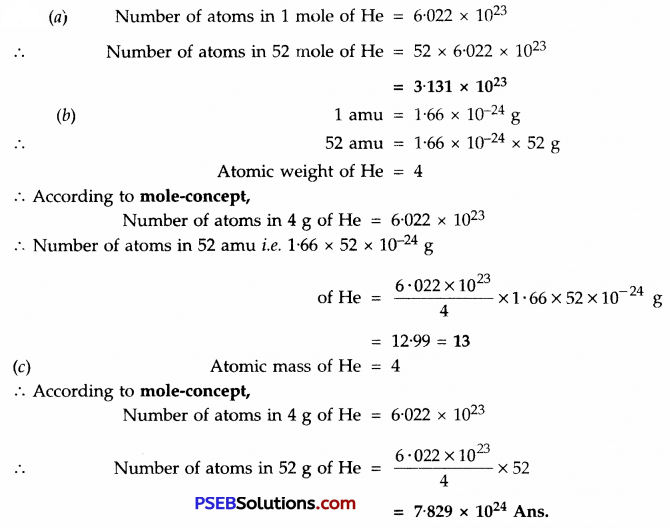Question 1.
When did Indian philosophers thought of division of matter?
Around 500 B.C.

Question 2.
What was the view of Maharishi Kanad regarding the division of matter?
The smallest particle of matter will be indivisible.Question 3.
What was the name of smallest indivisible particle by Kanad?
Atom.

Question 4.
Which two Greek philosophers considered smallest particle as indivisible?
Democritus and Leucippus.

Question 5.
What is the ratio by mass of H and O in water?
1 : 8.

Question 6.
What is the ratio by mass of N and H in NH3?
14 : 3,

Question 7.
What is Prout’s law of constant composition?
A pure chemical compound is always made up of same elements combined together in the same fixed ratio by mass.

Question 8.
Who gave Atomic theory?
John Dalton.

Question 9.
When did John Dalton gave atomic theory?
In 1808.

Question 10.
What are the units used to e×press the atomic radius?
Nanometre (nm).Question 11.
What is the relationship between m and nm?
1 nm = 10-9m.

Question 12.
What is the building block of all matter?
Atom.

Question 13.
Who was the first scientist which used symbols for elements?
Dalton.

Question 14.
What was special about symbols of elements given by Berzelius?
The symbols of elements were represented by one or two letters of the name of the element.

Question 15.
What were the units used for e×pressing atomic masses of elements initially?
Initially 1 /16th of mass of an atom of naturally occuring oxygen (016-isotope) was taken as unit.

Question 16.
When was C12 isotope universally accepted as standard reference for expressed atomic masses?
In 1961.

Question 17.
Which unit is used now-a-days to e×press atomic masses?
It is 1 /12th of mass of an atom of carbon (C12 – isotope).

Question 18.
Name the smallest particle of an element or compound which can exist freely?
Molecule.Question 19.
What is obtained when the atoms of the same element combine?
Molecule.

Question 20.
How many atoms are present in oxygen molecule.
Two, hence, it is called diatomic molecule (O2).

Question 21.
Give four examples of diatomic molecules.
H2, O2, N2 and Cl2.

Question 22.
Give one example of tetra-atomic molecule.
Phosphorous, (P4).

Question 23.
Give one example sample of octa-atomic molecule.
Sulphur, (S8).

Question 24.
What is produced when three atoms of oxygen combine.
Ozone (O3).

Question 25.
Name isotopes of carbon.
C12, C13 and C14.Question 26.
What is the ratio by mass of C and O in carbon dioxide?
3 : 8

Question 27.
What is the ratio by number between the atoms of H and O in water?
2 : 1

Question 28.
Define an ion.
It is an atom or group of atoms having charge (positive or negative).

Question 29.
What is a cation?
It is an ion having positive charge.

Question 30.
What is an anion?
It is an ion having negative charge.

Question 31.
What is the ratio by mass of Ca nd O in calcium oxide (CaO)?
5 : 2Question 32.
What is the ratio by mass of Mg and S in magnesium sulphate?
3 : 4

Question 33.
What is the ratio by mass of Na and Cl in sodium chloride (NaCl)?
23 : 35.5

Question 34.
Define chemical formula?
It is the symbolic representation of a molecule of a substance.

Question 35.
Define valency.
It is the combining capacity of an atom of the element.

Question 36.
What are diatomic molecules?
These are made up of two atoms made up of same or different elements.

Question 37.
Define molecular mass of a subtance?
It is the average relative mass of one molecule of substance as compared to the mass of one atom of carbon, (C12-isotope).Question 38.
Which unit is used for expressing molecular masses?
a.m.u.

Question 39.
What is the new name given to a.m.u. by IUPAC?
U (Unified).

Question 40.
Name the elements present in water (H2O).
Hydrogen and oxygen.

Question 41.
What is one mole of an element?
It is the number of an element whose mass in grams is numerically equal to its atomic mass e×pressed in a.m.u. or 1 gram atom of an element represents one mole.

Question 42.
What is Avogardro’s number?
Avogardro’s number contains 6.022 × 1023 particles.

Question 43.
By No or NA.Question 44.
In what ratio elements combine to form a compound?
In a fixed ratio by mass.

Question 45.
Which scientist gave law of conservation of mass?
Russian scientist Lavoisier in 1756.

Question 46.
Which scientist gave law of constant composition?
French scientist Joseph L. Proust in 1799.

Question 47.
What does a.m.u. represent?
It is the unit used to express atomic masses.

Question 48.
Which isotope of carbon is used as a standard reference to express atomic masses?
C12 – isotope.

Question 49.
Most of the elements have fractional atomic masses, what does it show?
This indicates the existence of isotopes of an element.

Question 50.
What is molar mass?
It is the mass of 1 mole of a substance in grams.

Question 51.
Define gram atomic mass.
It is the mass of 1 mole of atoms (6.022 × 1023) in grams.Question 52.
How many atoms are present in 1 gram atom of an element?
6.022 × 1023.

Question 53.
Do 1 mole of sodium and calcium have different number of atoms?
No, they have same number of atoms.

Question 54.
Lead nitrate solution and sodium chloride solution taken in separate beakers. The beakers were weighed and mixed in one beaker and the beaker was weighed again. Does weight remains unchanged or not? On what principle, the answer is based upon?
The weight remains unchanged. It is based upon law of conservation of mass.

Question 55.
28 g of magnesium oxide is produced by the combination of 12 g of magnesium and 16 g of oxygen. Which law is illustrated by this data?
Law of conservation of mass.

Question 56.
Do 1 mole of Na and 02 have same mass?
No, 1 mole of Na has mass = 23 g
1 mole of O2 has mass = 32 g

Question 57.
1 mole represents particles …………. .
6.022 × 1023.

Question 58.
The molar mass of NH3 is
17 g.Question 59.
An element Z has a valency of 3. Write down the formula of its oxide?
Z2O3.

Question 60.
How many atoms are present in 0.012 kg of C12 – isotope? What is this number called?
6.022 × 1023 atoms. This number is called Avogadro’s number.

Question 61.
Write down the formula of compound made up of Al3+ and [latetx]\mathrm{SO}_{4}^{2-}[/latex].
Al2 (SO4)3

Question 62.
How many molecules are present in 9 g of water?
9/18 × 6.0222 × 1023 = 3.011 × 1023 molecules.

Question 63.
What is the molecular mass of HN03. (Atomic masses, H = lu, N = 14u, 0 = 16u)